# Uncertainties, Graphing, and the Vernier Caliper

 Copyright July 1, 2000 Vern Lindberg

## Contents

1. Systematic versus Random Errors
2. Determining Random Errors (a) Instrument Limit of Error, least count  (b) Estimation  (c)  Average Deviation
(d)  Conflicts (e) Standard Error in the Mean
3. What does uncertainty tell me?  Range of possible values
4.  Relative and Absolute error
5.  Propagation of errors  (a) add/subtract  (b)  multiply/divide  (c) powers  (d) mixtures of +-*/ (e) other functions
7.  Significant figures
8.  Problems to try
9.  Glossary of terms (all terms that are bold face and underlined)

### Part III The Vernier Caliper

 In this manual there will be problems for you to try. They are highlighted in yellow, and have answers. There are also examples highlighted in green.

## 5. Propagation of Errors, Basic Rules

Suppose two measured quantities x and y have uncertainties, Dx and Dy, determined by procedures described in previous sections: we would report (x ± Dx), and (y ± Dy). From the measured quantities a new quantity, z, is calculated from x and y. What is the uncertainty, Dz, in z? For the purposes of this course we will use a simplified version of the proper statistical treatment. The formulas for a full statistical treatment (using standard deviations) will also be given. The guiding principle in all cases is to consider the most pessimistic situation. Full explanations are covered in statistics courses.

The examples included in this section also show the proper rounding of answers, which is covered in more detail in Section 6.  The examples use the propagation of errors using average deviations.

### (a) Addition and Subtraction: z = x + y     or    z = x - y

 Derivation: We will assume that the uncertainties are arranged so as to make z as far from its true value as possible. Average deviations  Dz = |Dx| + |Dy| in both cases With more than two numbers added or subtracted we continue to add the uncertainties.

 Using simpler average errors Using standard deviationsEq. 1aEq. 1b

Example:  w = (4.52 ± 0.02) cm, x = ( 2.0 ± 0.2) cm, y = (3.0 ± 0.6) cm. Find z = x + y - w and its uncertainty.

z = x + y - w = 2.0 + 3.0 - 4.5 = 0.5 cm

 Dz = Dx + Dy + Dw = 0.2 + 0.6 + 0.02 = 0.82  rounding to  0.8 cm So z = (0.5 ± 0.8) cm Solution with standard deviations, Eq. 1b, Dz = 0.633 cm z = (0.5 ± 0.6) cm

Notice that we round the uncertainty to one significant figure and round the answer to match.

 For multiplication by an exact number, multiply the uncertainty by the same exact number.

 Example:  The radius of a circle is x = (3.0 ± 0.2) cm. Find the circumference and its uncertainty. C = 2 p x = 18.850 cm   DC = 2 p Dx = 1.257 cm (The factors of 2 and p are exact) C = (18.8 ± 1.3) cm We round the uncertainty to two figures since it starts with a 1, and round the answer to match.

Example:  x = (2.0 ± 0.2) cm, y = (3.0 ± 0.6) cm. Find z = x - 2y and its uncertainty.

 z = x - 2y = 2.0 - 2(3.0) = -4.0 cm Dz = Dx + 2 Dy = 0.2 + 1.2 = 1.4 cm So  z = (-4.0 ± 1.4) cm. Using Eq 1b, z = (-4.0 ± 0.9) cm.

The 0 after the decimal point in 4.0 is significant and must be written in the answer. The uncertainty in this case starts with a 1 and is kept to two significant figures. (More on rounding in Section 7.)### (b) Multiplication and Division: z = x y    or    z = x/y

 Derivation: We can derive the relation for multiplication easily. Take the largest values for x and y, that is z + Dz = (x + Dx)(y + Dy) = xy + x Dy + y Dx + Dx Dy Usually Dx << x and Dy << y so that the last term is much smaller than the other terms and can be neglected. Since z = xy, Dz = y Dx + x Dy which we write more compactly by forming the relative error, that is the ratio of Dz/z, namelyThe same rule holds for multiplication, division, or combinations, namely add all the relative errors to get the relative error in the result.

 Using simpler average errors Using standard deviationsEq. 2a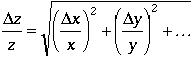Eq.2b

Example:  w = (4.52 ± 0.02) cm, x = (2.0 ± 0.2) cm.  Find z = w x and its uncertainty.

z = w x = (4.52) (2.0) = 9.04So Dz = 0.1044 (9.04) = 0.944  which we round to  0.9, z = (9.0 ± 0.9). Using Eq. 2b we get  Dz = 0.905and  z = (9.0 ± 0.9).

The uncertainty is rounded to one significant figure and the result is rounded to match. We write 9.0rather than 9since the 0 is significant.

Example:  x = ( 2.0 ± 0.2) cm, y = (3.0 ± 0.6) sec Find z = x/y.

 z = 2.0/3.0 = 0.6667 cm/s. So Dz = 0.3 (0.6667 cm/sec) = 0.2 cm/sec z = (0.7 ± 0.2) cm/sec Using Eq. 2b we get  z = (0.67 ± 0.15) cm/sec

Note that in this case we round off our answer to have no more decimal places than our uncertainty.### (c) Products of powers:.

The results in this case are

 Using simpler average errors Using standard deviations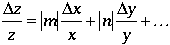Eq. 3aEq.3b

Example:  w = (4.52 ± 0.02) cm, A = (2.0 ± 0.2), y = (3.0 ± 0.6) cm. Find.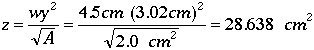The second relative error, (Dy/y), is multiplied by 2 because the power of y is 2.  The third relative error, (DA/A), is multiplied by 0.5 since a square root is a power of one half. So Dz = 0.49 (28.638) = 14.03which we round to 14z = (29 ± 14)Using Eq. 3b, z=(29 ± 12)Because the uncertainty begins with a 1, we keep two significant figures and round the answer to match.### (d)  Mixtures of multiplication, division, addition, subtraction, and powers.

If z is a function which involves several terms added or subtracted we must apply the above rules carefully.  This is best explained by means of an example.

Example:  w = (4.52 ± 0.02) cm, x = (2.0 ± 0.2) cm, y = (3.0 ± 0.6) cm. Find z = w x +y^2

z = wx +y^2 = 18.0First we compute v = wx as in the example in (b) to get v = (9.0 ± 0.9). Next we compute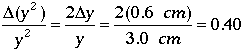Finally, we compute Dz = Dv + D(y^2) = 0.9 + 3.6 = 4.5rounding to 4Hence z = (18 ± 4). We have v = wx = (9.0 ± 0.9) cm.  The calculation of the uncertainty inis the same as that shown to the left. Then from Eq. 1b  Dz =  3.7z = (18 ± 4).### (e) Other Functions: e.g.. z = sin x. The simple approach.

For other functions of our variables such as sin(x) we will not give formulae. However you can estimate the error in z = sin(x) as being the difference between the largest possible value and the average value. and use similar techniques for other functions.
Thus

D(sin x) = sin(x + Dx) - sin(x)

 Example:  Consider S = x cos (q) for x = (2.0 ± 0.2) cm, q = 53 ± 2 °. Find S and its uncertainty. S = (2.0 cm) cos 53° = 1.204 cm To get the largest possible value of S we would make x larger, (x + Dx) = 2.2 cm, and q smaller, (q - Dq) = 51°. The largest value of S, namely (S + DS), is (S + DS) = (2.2 cm) cos 51° = 1.385 cm. The difference between these numbers is DS = 1.385 - 1.204 = 0.181 cm  which we round to 0.18 cm. Then S = (1.20 ± 0.18) cm.### (f) Other Functions: Getting formulas using partial derivatives

The general method of getting formulas for propagating errors involves the total differential of a function. Suppose that z = f(w, x, y, ...) where the variables w, x, y, etc. must be independent variables!

The total differential is thenWe treat the dw = Dw as the error in w, and likewise for the other differentials, dz, dx, dy, etc. The numerical values of the partial derivatives are evaluated by using the average values of w, x, y, etc. The general results are

 Using simpler average errors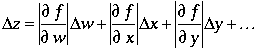Eq. 4a. Using standard deviationsEq. 4b

Example: Consider S = x cos (q) for x = (2.0 ± 0.2) cm, q = (53 ± 2) °= (0.9250 ± 0.0035) rad. Find S and its uncertainty. Note: the uncertainty in angle must be in radians!

S = 2.0 cm cos 53° = 1.204 cm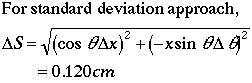Hence S = (1.20 ± 0.13) cm (using average deviation approach) or S = (1.20 ± 0.12) cm (using standard deviation approach.)## 6. Rounding off answers in regular and scientific notation.

In the above examples we were careful to round the answers to an appropriate number of significant figures. The uncertainty should be rounded off to one or two significant figures. If the leading figure in the uncertainty is a 1, we use two significant figures, otherwise we use one significant figure. Then the answer should be rounded to match.

 Example Round off z = 12.0349 cm and Dz = 0.153 cm. Since Dz begins with a 1, we round off Dz to two significant figures: Dz = 0.15 cm. Hence, round z to have the same number of decimal places: z = (12.03 ± 0.15) cm.

When the answer is given in scientific notation, the uncertainty should be given in scientific notation with the same power of ten. Thus, if

z = 1.43 x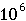s and Dz = 2 xs,

we should write our answer as

z = (1.43± 0.02) xs.

This notation makes the range of values most easily understood. The following is technically correct, but is hard to understand at a glance.

z = (1.43 x± 2 x) s. Don't write like this!

 Problem:  Express the following results in proper rounded form, x ± Dx. (i) m = 14.34506 grams, Dm = 0.04251 grams. (ii) t = 0.02346 sec, Dt = 1.623 x 10-3sec. (iii) M = 7.35 x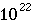kg DM = 2.6 xkg. (iv) m = 9.11 xkg Dm = 2.2345 xkg    Answer## 7. Significant Figures

The rules for propagation of errors hold true for cases when we are in the lab, but doing propagation of errors is time consuming. The rules for significant figures allow a much quicker method to get results that are approximately correct even when we have no uncertainty values.

A significant figure is any digit 1 to 9 and any zero which is not a place holder. Thus, in 1.350 there are 4 significant figures since the zero is not needed to make sense of the number. In a number like 0.00320 there are 3 significant figures --the first three zeros are just place holders. However the number 1350 is ambiguous. You cannot tell if there are 3 significant figures --the 0 is only used to hold the units place --or if there are 4 significant figures and the zero in the units place was actually measured to be zero.

How do we resolve ambiguities that arise with zeros when we need to use zero as a place holder as well as a significant figure? Suppose we measure a length to three significant figures as 8000 cm. Written this way we cannot tell if there are 1, 2, 3, or 4 significant figures. To make the number of significant figures apparent we use scientific notation, 8 x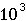cm (which has one significant figure), or 8.00 xcm (which has three significant figures), or whatever is correct under the circumstances.

We start then with numbers each with their own number of significant figures and compute a new quantity. How many significant figures should be in the final answer? In doing running computations we maintain numbers to many figures, but we must report the answer only to the proper number of significant figures.

In the case of addition and subtraction we can best explain with an example. Suppose one object is measured to have a mass of 9.9 gm and a second object is measured on a different balance to have a mass of 0.3163 gm. What is the total mass? We write the numbers with question marks at places where we lack information. Thus 9.9???? gm and 0.3163? gm. Adding them with the decimal points lined up we see

09.9????
00.3163?
10.2???? = 10.2 gm.

In the case of multiplication or division we can use the same idea of unknown digits. Thus the product of 3.413? and 2.3? can be written in long hand as

3.413?
2.3?
?????
10239?
6826?
7.8????? = 7.8

The short rule for multiplication and division is that the answer will contain a number of significant figures equal to the number of significant figures in the entering number having the least number of significant figures. In the above example 2.3 had 2 significant figures while 3.413 had 4, so the answer is given to 2 significant figures.

It is important to keep these concepts in mind as you use calculators with 8 or 10 digit displays if you are to avoid mistakes in your answers and to avoid the wrath of physics instructors everywhere.  A good procedure to use is to use use all digits (significant or not) throughout calculations, and only round off the answers to appropriate "sig fig."

 Problem:  How many significant figures are there in each of the following?    Answer (i) 0.00042   (ii) 0.14700   (ii) 4.2 x(iv) -154.090 x## 8. Problems on Uncertainties and Error Propagation.

Try the following problems to see if you understand the details of this part . The answers are at the end.

(a) Find the average and the average deviation of the following measurements of a mass.

4.32, 4.35, 4.31, 4.36, 4.37, 4.34 grams.

(b) Express the following results in proper rounded form, x ± Dx.

(i) m = 14.34506 grams, Dm = 0.04251 grams.
(ii) t = 0.02346 sec, Dt = 1.623 x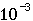sec.
(iii) M = 7.35 xkg DM = 2.6 xkg.
(iv) m = 9.11 xkg Dm = 2.2345 xkg

(c) Are the following numbers equal within the expected range of values?

(i) (3.42 ± 0.04) m/s and 3.48 m/s?
(ii) (13.106 ± 0.014) grams and 13.206 grams?
(iii) (2.95 ± 0.03) xm/s and 3.00 xm/s

(d) Calculate z and Dz for each of the following cases.

(i) z = (x - 2.5 y + w) for x = (4.72 ± 0.12) m, y = (4.4 ± 0.2) m, w = (15.63 ± 0.16) m.
(ii) z = (w x/y) for w = (14.42 ± 0.03) m/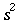, x = (3.61 ± 0.18) m, y = (650 ± 20) m/s.
(iii) z =for x = (3.55 ± 0.15) m.
(iv) z = v (xy + w) with v = (0.644 ± 0.004) m, x = (3.42 ± 0.06) m, y = (5.00 ± 0.12) m, w =    (12.13 ± 0.08).
(v) z = A sin y for A = (1.602 ± 0.007) m/s, y = (0.774 ± 0.003) rad.

(e) How many significant figures are there in each of the following?

(i) 0.00042   (ii) 0.14700   (ii) 4.2 x(iv) -154.090 x 10-27

(f) I measure a length with a meter stick which has a least count of 1 mm I measure the length 5 times with  results in mm of 123, 123, 124, 123, 123 mm. What is the average length and the uncertainty in length?(a) (4.342 ± 0.018) grams

(b)  i) (14.34 ± 0.04) grams      ii) (0.0235 ± 0.0016) sec or (2.35 ± 0.16) xsec
iii) (7.35 ± 0.03) xkg   iv) (9.11 ± 0.02) xkg

(c) Yes for (i) and (iii), no for (ii)

(d) i) (9.4 ± 0.8) m   ii) (0.080 ± 0.007) m/s   iii) (45 ± 6)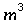iv) 18.8 ± 0.6)v) (1.120 ± 0.008 m/s

(e) i) 2   ii) 5   iii) 2   iv) 6

(f) (123 ± 1) mm (I used the ILE = least count since it is larger than the average deviation.)

## 9. Glossary of Important Terms

 Term Brief Definition Absolute error The actual error in a quantity, having the same units as the quantity. Thus if  c = (2.95 ± 0.07) m/s, the absolute error is 0.07 m/s. See Relative Error. Accuracy How close a measurement is to being correct. For gravitational acceleration near the earth, g = 9.7 m/s2 is more accurate than g = 9.532706 m/s2. See Precision. Average When several measurements of a quantity are made, the sum of the measurements divided by the number of measurements. Average Deviation The average of the absolute value of the differences between each measurement and the average. See Standard Deviation. Confidence Level The fraction of measurements that can be expected to lie within a given range. Thus if m = (15.34 ± 0.18) g, at 67% confidence level, 67% of the measurements lie within (15.34 - 0.18) g and (15.34 + 0.18) g. If we use 2 deviations (±0.36 here) we have a 95% confidence level. Deviation A measure of range of measurements from the average. Also called error oruncertainty. Error A measure of range of measurements from the average. Also called deviation or uncertainty. Estimated Uncertainty An uncertainty estimated by the observer based on his or her knowledge of the experiment and the equipment. This is in contrast to ILE, standard deviation or average deviation. Gaussian Distribution The familiar bell-shaped distribution. Simple statistics assumes that random errors are distributed in this distribution. Also called Normal Distribution. Independent Variables Changing the value of one variable has no effect on any of the other variables. Propagation of errors assumes that all variables are independent. Instrument Limit  of Error (ILE) The smallest reading that an observer can make from an instrument. This is generally smaller than the Least Count. Least Count The size of the smallest division on a scale. Typically the ILE equals the least count or 1/2 or 1/5 of the least count. Normal Distribution The familiar bell-shaped distribution. Simple statistics assumes that random errors are distributed in this distribution. Also called Gaussian Distribution. Precision The number of significant figures in a measurement. For gravitational acceleration near the earth, g = 9.532706 m/s2 is more precise than g = 9.7 m/s2. Greater precision does not mean greater accuracy! See Accuracy. Propagation of Errors Given independent variables each with an uncertainty, the method of determining an uncertainty in a function of these variables. Random Error Deviations from the "true value" can be equally likely to be higher or lower than the true value. See Systematic Error. Range of Possible True Values Measurements give an average value, and an uncertainty, Dx. At the 67% confidence level the range of possible true values is from - Dx  to + Dx. See Confidence Level . Relative Error The ratio of absolute error to the average, Dx/x. This may also be called percentage error or fractional uncertainty. See Absolute Error. Significant Figures All non-zero digits plus zeros that do not just hold a place before or after a decimal point. Standard Deviation The statistical measure of uncertainty. See Average Deviation. Standard Error in the Mean An advanced statistical measure of the effect of large numbers of measurements on the range of values expected for the average (or mean). Systematic Error A situation where all measurements fall above or below the "true value". Recognizing and correcting systematic errors is very difficult. Uncertainty A measure of range of measurements from the average. Also called deviation or error.
Send any comments or corrections to Vern Lindberg.